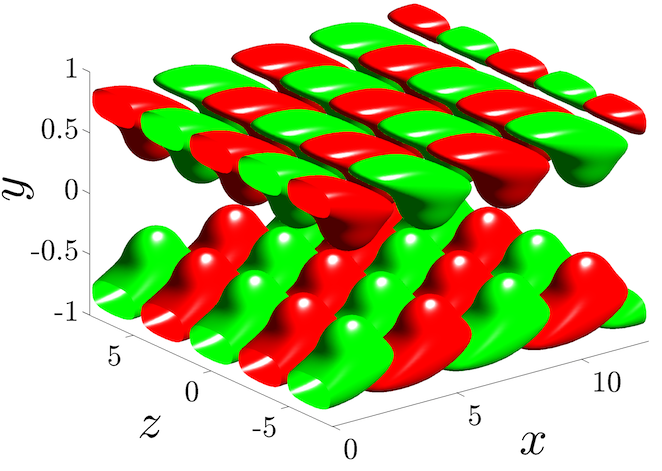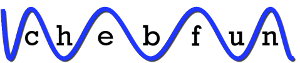# Singular Value Decomposition and Frequency Responses of PDEs in ChebfunOriginally released in December 2011; Updated in May 2013 Matlab Files Download all Matlab files Presentation 2012 SIAM Annual Meeting TalkThis work utilizes Chebfun V4.2.2889, released in April 2013. Chebfun is available at http://www.maths.ox.ac.uk/chebfun/

## Introduction

In many physical systems there is a need to examine the effects of exogenous disturbances on the variables of interest. The frequency response analysis represents an effective means for quantifying the system's performance in the presence of a stimulus, and it characterizes the steady-state response of a stable system to persistent harmonic forcing. For infinite dimensional systems, the principal singular value of the frequency response operator quantifies the largest amplification from the input forcing to the desired output at each frequency. Furthermore, the associated left and right principal singular functions identify the spatial distributions of the output (that exhibits this largest amplification) and the input (that has the strongest influence on the system's dynamics), respectively.

We have developed mathematical framework and computational tools for calculating frequency responses of linear time-invariant partial differential equations (PDEs) in which an independent spatial variable belongs to a compact interval. In conventional studies this computation is done numerically using spatial discretization of differential operators in the evolution equation. Our method avoids this by recasting the frequency response operator as a two point boundary value problem and using state-of-the-art automatic spectral collocation techniques for solving the resulting eigenvalue problems with accuracy comparable to machine precision. The algorithm is based on transforming the two point boundary value problem in differential form into an equivalent integral representation. Our approach has two advantages over currently available schemes: first, it avoids numerical instabilities encountered in systems with differential operators of high order and, second, it alleviates difficulty in implementing boundary conditions. We refer the users to [Lieu & Jovanovic, J. Comput. Phys. 2013] for a detailed explanation of the method.

Even though we confine our attention to computation of the frequency responses for PDEs, the developed framework allows users to employ Chebfun as a tool for determining singular value decomposition of compact operators that admit two point boundary value representations. In particular, our approach paves the way for overloading Matlab's command svds, from matrices to compact operators.

We have developed the following easy-to-use Matlab function (an m-file),

svdfr.m

which takes the system's coefficients and boundary condition matrices as inputs and returns the desired number of left (or right) singular pairs as the output. The coefficients and boundary conditions of the adjoint systems are automatically implemented within the code. Thus, the burden of finding the adjoint operators and corresponding boundary conditions is removed from the user.

```help svdfr
```
```  ========================================================================
[Sfun,Sval] = svdfr(A0,B0,C0,Wa0,Wb0,LRfuns,Nsigs)

Given a two point boundary value representation of the frequency response
operator

{ A0*phi = B0*d,
T:  { u = C0*phi,
{ 0 = Wa0*phi(a) + Wb0*phi(b),

solve the eigenvalue problem

T*Ts*Sfun = Sval*Sfun, or Ts*T*Sfun = Sval*Sfun,

where Ts is the adjoint of the frequency response operator T

{ A0s*psi = B0s*f,
Ts:  { g = C0s*psi,
{ 0 = Wa0s*psi(a) + Wb0s*psi(b).

Inputs:

LRfuns  = 1     --> solve for left singular functions: T*Ts
--> determine spatial profile of the output
LRfuns  = 0     --> solve for right singular functions: Ts*T
--> determine spatial profile of the input

Nsigs --> number of desired singular values (default: Nsigs = 1)

Outputs:

Sval    --> singular values of T arranged in descending order
Sfun    --> singular functions associated with Sval

Written by: Binh Lieu

Reference:

B. K. Lieu and M. R. Jovanovic
"Computation of frequency responses for linear time-invariant PDEs on a compact interval"
J. Comput. Phys., vol. 250, pp. 246-269, 2013.

========================================================================

```